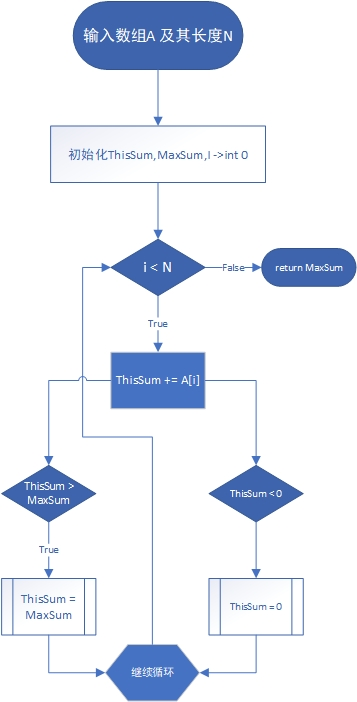Parksi

# 在线处理：最大子列和问题

In:列表 A[]，列表长度N
Out:列表A的最大子列和

## 算法实现

int MaxSubaseqSum(int A[], int N)
/* 求最大连续子列和 最优算法 “在线算法” */
{
int ThisSum, MaxSum;
int i;
ThisSum = MaxSum = 0;
for (i = 0; i < N; i++) {
ThisSum += A[i];
if (ThisSum > MaxSum)
MaxSum = ThisSum;
else if (ThisSum < 0)
ThisSum = 0;
}
return MaxSum;
}


## 分析

T(N) = O(N)

### 原理

• 子列和为负数时无法令当前子列与下一个数的和变大

### 算法流程

1. 从左向右读取数组
2. 从第一个数加起，如果子列和为正且大于最大子列和，则更新最大子列和，反之令当前子列和为0，继续读入